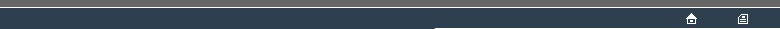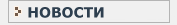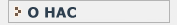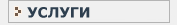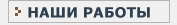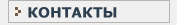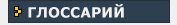СТАТИСТИЧЕСКИЙ В данном разделе Вы найдете все интересующие Вас термины и определения, связанные с статистикой и статистическими исследованиями.КЛИНИЧЕСКИЕ ИССЛЕДОВАНИЯ Необходимый перечень терминов и определений, используемых при проведении клинических испытанийB BERNOUILLI PROCESS [()] This is the simplest probability model - a single trial between two possible outcomes such as a coin toss. The distribution depends upon a single parameter,'p', representing the probability attributed to one defined outcome out of the two possible outcomes. Also see : BINOMIAL DISTRIBUTION, POISSON PROCESS. BETA Also known as TYPE-2 ERROR, BETA is the complement to POWER : BETA = (1-POWER). This is the probability that a statistical test will generate a false-negative error : failing to assert a defined pattern of deviation from a null pattern in circumstances where the defined pattern exists. Conventional methodology for statistical testing is to set in advance a NOMINAL ALPHA CRITERION LEVEL - the corresponding level for BETA will depend upon the NOMINAL ALPHA CRITERION LEVEL and upon further considerations including the strength of the pattern in the data and the sample size. Interest is generally in the RELATIVE POWER of different tests rather than in an absolute value. It is questionable whether the concept of BETA error is properly applicable without considering the concept of sampling from a population, which is separate from the concerns of this Glossary. Applicability of this reasoning is also closely bound up with the choice of TEST STATISTIC. Also see : ERROR TYPES. BINOMIAL DISTRIBUTION This is a special case of the MULTINOMIAL DISTRIBUTION where the number of possible outcomes is 2. It is the distribution of outcomes expected if a certain number of independent trials are undertaken of a single BERNOUILLI PROCESS (e.g. multiple tosses of a coin, or tosses of several coins with identical characteristics). The distribution depends upon the single parameter,'p', of the corresponding BERNOULLI PROCESS and upon the number of trials, 'n'. An alternative characterisation is as the outcome of two separate POISSON PROCESSEs with separate rate parameters. BINOMIAL TEST This is a statistical test referring to a repeated binary process such as would be expected to generate outcomes with a BINOMIAL DISTRIBUTION. A value for the parameter 'p' is hypothesised (null hypothesis) and the difference of the actual value from this is assessed as a value of ALPHA. Also see : EXACT BINOMIAL TEST. BOOTSTRAP [()] This is a form of RANDOMISATION TEST which is one of the alternatives to EXHAUSTIVE RE-RANDOMISATION. The BOOTSTRAP scheme involves generating subsets of the data on the basis of random sampling with replacements as the data are sampled. Such resampling provides that each datum is equally represented in the randomisation scheme; however, the BOOTSTRAP procedure has features which distinguish it from the procedure of a MONTE-CARLO TEST. The distinguishing features of the BOOTSTRAP procedure are concerned with over-sampling - there is no constraint upon the number of times that a datum may be represented in generating a single resampling subset; the size of the resampling subsets may be fixed arbitrarily independently of the parameter values of the EXPERIMENTAL DESIGN and may even exceed the total number of data. The positive motive for BOOTSTRAP resampling is the general relative ease of devising an appropriate resampling ALGORITHM(1) when the EXPERIMENTAL DESIGN is novel or complex. A negative aspect of the BOOTSTRAP is that the form of the resampling distribution with prolonged resampling converges to a form which depends not only upon the data and the TEST STATISTIC, but also upon the BOOTSTRAP resampling subset size - thus the resampling distribution should not be expected to converge to the GOLD STANDARD(1) form of the EXACT TEST as is the case for MONTE-CARLO resampling. An effective necessity for the BOOTSTRAP procedure is a source of random codes or an effective PSEUDO-RANDOM generator. BRANCH-AND-BOUND Exploration of a RANDOMISATION DISTRIBUTION in such a way as to anticipate the effect of the next RANDOMISATION(3) relative to the present RANDOMISATION(3). This allows selective search of particular zones of a RANDOMISATION DISTRIBUTION; in the context of a RANDOMISATION TEST such selective search may be concerned with the TAIL of the RANDOMISATION DISTRIBUTION. Also see : RANOMISATION TEST(1).B
BERNOUILLI PROCESS
BETA
BINOMIAL DISTRIBUTION
BINOMIAL TEST
BOOTSTRAP
BRANCH-AND-BOUNDНовости   О нас   Услуги   Наши работы   Статьи   Контакты   Глоссарий
Статистическая помощь! © 2005 - 2023 • Защита авторских прав